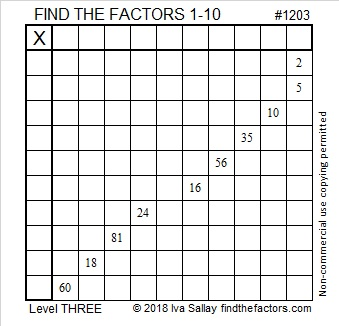# 1203 and Level 3

At the top of this level 3 puzzle are two clues that will tell you where to put three of the factors needed to solve the puzzle. After you find those three clues work down looking at the clues cell by cell until you have the entire puzzle solved.Print the puzzles or type the solution in this excel file: 10-factors-1199-1210

Here are a few facts about the number 1203:

• 1203 is a composite number.
• Prime factorization: 1203 = 3 × 401
• The exponents in the prime factorization are 1 and 1. Adding one to each and multiplying we get (1 + 1)(1 + 1) = 2 × 2 = 4. Therefore 1203 has exactly 4 factors.
• Factors of 1203: 1, 3, 401, 1203
• Factor pairs: 1203 = 1 × 1203 or 3 × 401
• 1203 has no square factors that allow its square root to be simplified. √1203 ≈ 34.68429Since 1203 is only made from three consecutive numbers (1, 2, 3) and zeros, it has to be divisible by 3.

1203 is the hypotenuse of a Pythagorean triple:
120-1197-1203 which is 3(40-399-401)